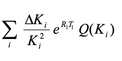## how to conduct vectorized summation in SAS/IML

Hi all,

I am building a formula which shows below by referring Sum a series in SASWhen I run,

``````start Sumcon(n);
i = 1:n;                    /* index of terms */
return( sum((deltak/k[i]##2)#exp(r[i]#T[i])#Q[i]) );  /* sum of terms */
finish;
n = T(1:68);
sum = j(nrow(n),1);     /* allocate a vector for the results */
do k = 1 to nrow(n);
sum[k] = Sumcon( n[k] );
end;``````

It returns ERROR: (execution) Matrix has not been set to a value.

where the variables k, r, t, Q are all (68,1) vectors, the deltak is a fixed value 5.

How can I achieve this formula?

Thanks a lot.

1 ACCEPTED SOLUTION

Accepted Solutions

## Re: how to conduct vectorized summation in SAS/IML

Here is how I would compute the sum. I am using 5-dimensional vectors instead of 68 elements:

``````proc iml;
/* Use n = 5 instead of 68.
Make up some fake values for the column vectors k, r, t, Q */
k = {1,2,3,4,5};
r = {1,2,3,4,5} / 5;
T = {1,2,3,4,5} / 10;
Q = {1,2,3,4,5} / 15;
deltak = 2;

v = deltak/k##2 # exp(r#T)#Q;
sum = sum(v);
``````
7 REPLIES 7

## Re: how to conduct vectorized summation in SAS/IML

ERROR: (execution) Matrix has not been set to a value.

Print out each of the matrices involved and see which one(s) do not have values.

--
Paige Miller

## Re: how to conduct vectorized summation in SAS/IML

Thanks for replying. However, every column is valued. Is it caused by incorrect use of the sum function?

## Re: how to conduct vectorized summation in SAS/IML

The vectors are not in scope inside the function. You either need to pass them in or use a GLOBAL statement. See "Understanding local and global variables in the SAS/IML language."

## Re: how to conduct vectorized summation in SAS/IML

Here is how I would compute the sum. I am using 5-dimensional vectors instead of 68 elements:

``````proc iml;
/* Use n = 5 instead of 68.
Make up some fake values for the column vectors k, r, t, Q */
k = {1,2,3,4,5};
r = {1,2,3,4,5} / 5;
T = {1,2,3,4,5} / 10;
Q = {1,2,3,4,5} / 15;
deltak = 2;

v = deltak/k##2 # exp(r#T)#Q;
sum = sum(v);
``````

## Re: how to conduct vectorized summation in SAS/IML

Why r, t, Q should divide by 5, 10, 15 respectively? how can I express this when read all var?

## Re: how to conduct vectorized summation in SAS/IML

Please take another look at Rick's post above, as I think it is the solution that you want. Ignore everything up to deltak=2, as this is artificial data to show that the solution works. Try to include the last 2 lines in your own code, and tell us what happens.

## Re: how to conduct vectorized summation in SAS/IML

The comment in my program says "Make up some fake values for the column vectors k, r, t, Q"